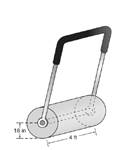Chapter 9.3, Problem 49EElementary Geometry For College St...

7th Edition
Alexander + 2 others
ISBN: 9781337614085

Solutions

Chapter
SectionElementary Geometry For College St...

7th Edition
Alexander + 2 others
ISBN: 9781337614085
Textbook Problem

A lawn roller in the shape of a right circular cylinder has a radius of length 18 in. and a length (height) of 4 ft. Find the area rolled during one complete revolution of the roller. Use the calculator value of π , and give the answer to the nearest square foot.To determine

To find:

The area rolled during one complete revolution of the roller.

Explanation

Given:

A lawn roller in the shape of a right circular cylinder has a radius of 18 in. and a length (height) of 4 ft.

Formula used:

The lateral area of the circular cylinder is L=2πrh

Calculation:

Let r be the radius and h be the altitude of the circular cylinder.

We have r=18 in

r=1812 ft=1.5 ft

We have r=1.5,h=4

Substitute the values in L=2πrh

Still sussing out bartleby?

Check out a sample textbook solution.

See a sample solution

The Solution to Your Study Problems

Bartleby provides explanations to thousands of textbook problems written by our experts, many with advanced degrees!

Get Started

In Exercises 516, evaluate the given quantity. log4116

Finite Mathematics and Applied Calculus (MindTap Course List)

In Exercises 2340, find the indicated limit. 40. limx3xx2+72x2x+3

Applied Calculus for the Managerial, Life, and Social Sciences: A Brief Approach

22. Let . Prove .

Elements Of Modern Algebra

Evaluate the integral. 35. /2/2x1+cos2xdx

Single Variable Calculus: Early Transcendentals